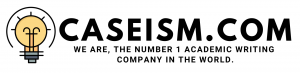# HEDGING NUMERICALS Case Solution & Answer

## HEDGING NUMERICALS Case Solution

e).

1. The hedge is selective since we hedging 100% of the total exposure.
2. The hedge is not perfect since the realized futures price is lower than the expected futures price.
3. The hedge is a short hedge since we are hedging the risk of the decline in the precious metal prices.

f).

I would say to the president that the hedge did not work because of the unexpected basis risk in the market due to the increased instability in the market.

Question 6

a).

A long position would be taken in order to hedge the risk of the rise in the price of palladium.

b).

The expected futures price would be: \$ 720 â€“ 2 = \$ 718 per troy ounce.

c).

The future basis would be:

F2 â€“ S2= Â 744 – 745 = -\$ 1 per troy ounce

The realized futures price would be:

F1 + Future basis: 720 + (-1) = \$ 719 per ounce

d).

The actual price is not equal to the quoted price because of the short basis position.

e).

The basis has weakened in this case.

Question 7

a).

A long position should be taken by the cooperative manager to hedge his corn purchase to hedge the risk of the rise in the price of the new harvest corn.

b).

Assuming the standard size of the corn futures contracts of 5000 bushels, the total number of the futures contracts needed would be: 100000/5000 = 20 contracts.

c).

The expected hedge price in the futures market would be: \$ 5.2 â€“ 0.3 = \$ 4.9 per bushel.

d).

The future basis would be:

F2 â€“ S2= 5.4 â€“ 5.2 = \$ 0.2 per bushel

The realized futures price would be:

F1 + Future basis: 5.2 + (0.2) = \$ 5.4

e).

The expected hedge price is not equal to the realized hedge price because of the change in the movement of the basis risk.

f).

Since, the basis risk has strengthened from -\$0.3 to \$0.2 therefore, there has been a difference in the expected and the realized price of corn.

Question 8

a).

A short position needs to be taken by the company for hedging the risk of the decrease in the price of the 1000 units of palladium. The first short position would be taken for the 500 units for the June sales and the second short position would be taken for the 500 units for December sales.

b).

The expected futures price which the mine should expect to get for its palladium sales would be:

For June: 773 + 3.25 = \$ 776.26 per troy ounce

For December: 775 + 3 = \$ 778 per troy ounce

c).

The realized hedge price for the lot sold in June would be as follows.

The future basis would be:

S2 â€“ F2 = 786 â€“ 783 = \$ 3 per troy ounce

The realized futures price would be:

F1 + Future basis: 773 + (3) = \$ 776 per troy ounce

d). The actual price is not equal to the quoted price because of the changes in the basis points. The movement of the basis risk shows that it has weakened.

e).

The realized hedge price for the lot sold in November would be as follows.

The future basis would be:

S2 â€“ F2 = 760 â€“ 763 = -\$ 3 per troy ounce

The realized futures price would be:

F1 + Future basis: 773 + (-3) = \$ 770 per troy ounce

f). The actual price is not equal to the quoted price because of the changes in the basis points. The movement of the basis risk shows that it has weakened.

Question 9

a).

In order to hedge his cash position, the builder needs to take a long position in the futures market.

b).

The expected hedge price of the builder is: 305 + 5 = \$ 310/MBF

c).

The realized hedge price for the builder would be as follows.

The future basis would be:

F2 â€“ S2= 320 – 315 = \$ 5 per MBF…………..

This is just a sample partial work. Please place the order on the website to get your own originally done case solution